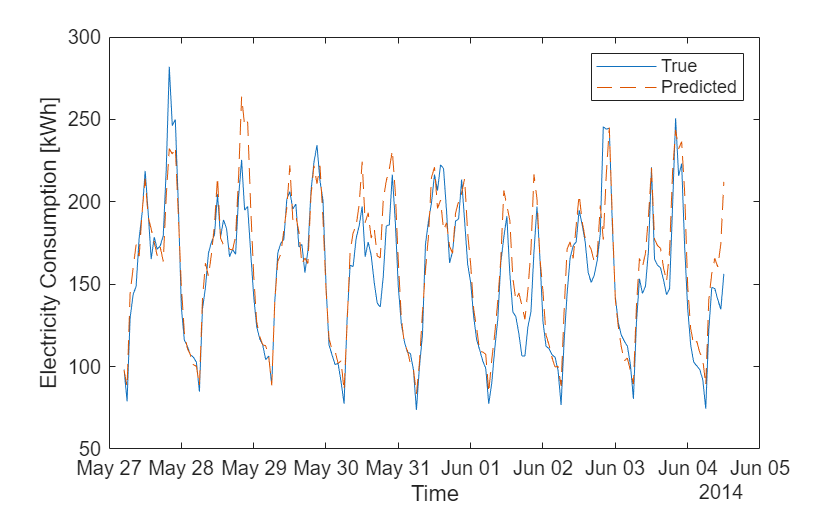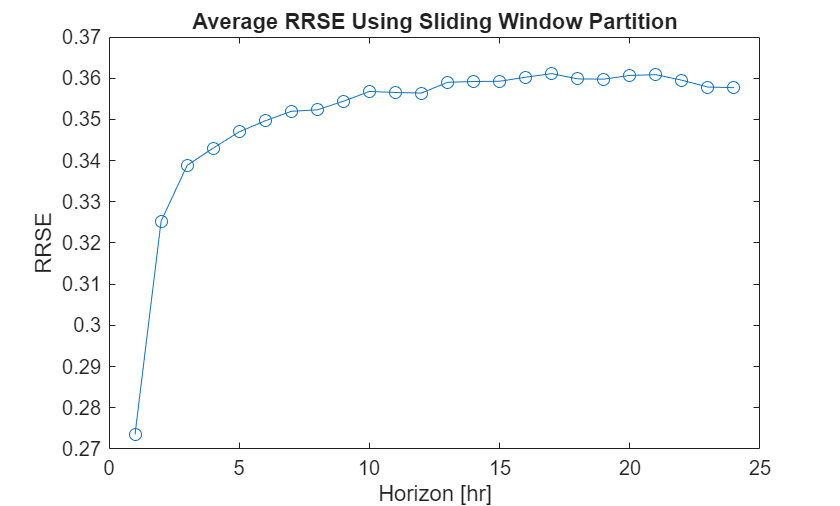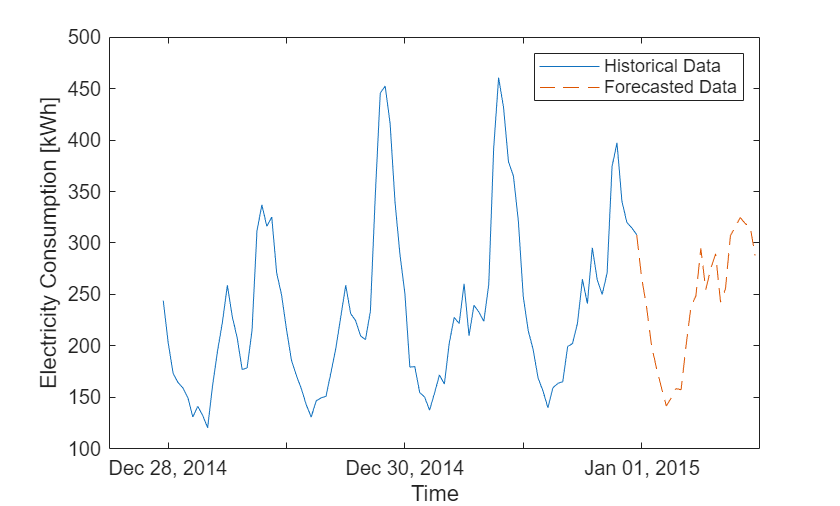# Time Series Forecasting Using Ensemble of Boosted Regression Trees

Perform single-step and multiple-step time series forecasting with an ensemble of boosted regression trees. Use different validation schemes, such as holdout, expanding window, and sliding window, to estimate the performance of the forecasting models.

At time t, you can train a direct forecasting model (predMdl) that predicts the value of a data point at a future time step $t+h$ (${\underset{}{\overset{ˆ}{y}}}_{t+h}$) by using the latest observations (${y}_{t},{y}_{t-1},...,{y}_{t-maxLag}$) and other observed variables at the current time (${X}_{t}$). That is,

${\underset{}{\overset{ˆ}{y}}}_{t+h}=predMdl\left({y}_{t},{y}_{t-1},...,{y}_{t-maxLag},{X}_{t}\right)$.

h is the look-ahead horizon used to train the model, and maxLag is the number of past observations used for forecasting. To forecast a data point at time $t+h+1$, train another model that uses $\mathit{h}+1$ as the look-ahead horizon.

This example shows how to:

• Create a single model that forecasts a fixed number of steps (24 hours) into the horizon. Use holdout validation and expanding window cross-validation to assess the performance of the model.

• Create multiple models for different look-ahead horizons (1-24 hours) as described in . Each of the 24 models forecasts a different hour into the horizon. Use holdout validation and sliding window cross-validation to assess the performance of the models.

• Create multiple models to forecast into the next 24 hours beyond the available data.

In this example, use electricity consumption data to create forecasting models. Load the data in `electricityclient.mat`, which is a subset of the ElectricityLoadDiagrams20112014 data set available in the UCI Machine Learning Repository . The original data set contains the electricity consumption (in kWh) of 321 clients, logged every 15 minutes from 2012 to 2014, as described in . The smaller `usagedata` timetable contains the hourly electricity consumption of the sixth client only.

`load electricityclient.mat`

Plot the electricity consumption of the sixth client during the first 200 hours. Overall, the electricity consumption of this client shows a periodicity of 24 hours.

```hrs = 1:200; plot(usagedata.Time(hrs),usagedata.Electricity(hrs)) xlabel("Time") ylabel("Electricity Consumption [kWh]")```Confirm that the values in `usagedata` are regular with respect to time by using the `isregular` function. For you to use past values of the data as features or predictors, your data must be regularly sampled.

`isregular(usagedata)`
```ans = logical 1 ```

Confirm that no values are missing in the time series by using the `ismissing` function.

`sum(ismissing(usagedata))`
```ans = 0 ```

If your data is not regularly sampled or contains missing values, you can use the `retime` function or fill the missing values. For more information, see Clean Timetable with Missing, Duplicate, or Nonuniform Times.

### Prepare Data for Forecasting

Before forecasting, reorganize the data. In particular, create separate time-related variables, lag features, and a response variable for each look-ahead horizon.

Use the date and time information in the `usagedata` timetable to create separate variables. Specifically, create `Month`, `Day`, `Hour`, `WeekDay`, `DayOfYear`, and `WeekOfYear` variables, and add them to the `usagedata` timetable. Let `numVar` indicate the number of variables in `usagedata`.

```usagedata.Month = month(usagedata.Time); usagedata.Day = day(usagedata.Time); usagedata.Hour = hour(usagedata.Time); usagedata.WeekDay = weekday(usagedata.Time); usagedata.DayOfYear = day(usagedata.Time,"dayofyear"); usagedata.WeekOfYear = week(usagedata.Time,"weekofyear"); numVar = size(usagedata,2);```

Normalize the time variables that contain more than 30 categories, so that their values are in the range –0.5 to 0.5. Specify the remaining time variables as categorical predictors.

```usagedata(:,["Day","DayOfYear","WeekOfYear"]) = normalize( ... usagedata(:,["Day","DayOfYear","WeekOfYear"]),range=[-0.5 0.5]); catPredictors = ["Month","Hour","WeekDay"];```

Create lag features to use as predictors by using the `lag` function. That is, create 23 new variables, `ElectricityLag1` through `ElectricityLag23`, where the lag number indicates the number of steps the `Electricity` data is shifted backward in time. Use the `synchronize` function to append the new variables to the `usagedata` timetable and create the `dataWithLags` timetable.

```dataWithLags = usagedata; maxLag = 23; for i = 1:maxLag negLag = lag(usagedata(:,"Electricity"),i); negLag.Properties.VariableNames = negLag.Properties.VariableNames + ... "Lag" + i; dataWithLags = synchronize(dataWithLags,negLag,"first"); end```

View the first few rows of the first three lag features in `dataWithLags`. Include the `Electricity` column for reference.

```head(dataWithLags(:,["Electricity","ElectricityLag1","ElectricityLag2", ... "ElectricityLag3"]))```
``` Time Electricity ElectricityLag1 ElectricityLag2 ElectricityLag3 ____________________ ___________ _______________ _______________ _______________ 01-Jan-2012 00:00:00 1056 NaN NaN NaN 01-Jan-2012 01:00:00 1363 1056 NaN NaN 01-Jan-2012 02:00:00 1240 1363 1056 NaN 01-Jan-2012 03:00:00 845 1240 1363 1056 01-Jan-2012 04:00:00 647 845 1240 1363 01-Jan-2012 05:00:00 641 647 845 1240 01-Jan-2012 06:00:00 719 641 647 845 01-Jan-2012 07:00:00 662 719 641 647 ```

Prepare the response variables for the look-ahead horizons 1 through 24. That is, create 24 new variables, `HorizonStep1` through `HorizonStep24`, where the horizon step number indicates the number of steps the `Electricity` data is shifted forward in time. Append the new variables to the `dataWithLags` timetable and create the `fullData` timetable.

```fullData = dataWithLags; maxHorizon = 24; for i = 1:maxHorizon posLag = lag(usagedata(:,"Electricity"),-i); posLag.Properties.VariableNames = posLag.Properties.VariableNames + ... "HorizonStep" + i; fullData = synchronize(fullData,posLag,"first"); end```

View the first few rows of the first two response variables in `fullData`. Include the `Electricity` column for reference.

```head(fullData(:,["Electricity","ElectricityHorizonStep1", ... "ElectricityHorizonStep2"]))```
``` Time Electricity ElectricityHorizonStep1 ElectricityHorizonStep2 ____________________ ___________ _______________________ _______________________ 01-Jan-2012 00:00:00 1056 1363 1240 01-Jan-2012 01:00:00 1363 1240 845 01-Jan-2012 02:00:00 1240 845 647 01-Jan-2012 03:00:00 845 647 641 01-Jan-2012 04:00:00 647 641 719 01-Jan-2012 05:00:00 641 719 662 01-Jan-2012 06:00:00 719 662 552 01-Jan-2012 07:00:00 662 552 698 ```

Remove the observations that contain `NaN` values after the preparation of the lag features and response variables. Note that the number of rows to remove depends on the `maxLag` and `maxHorizon` values.

```startIdx = maxLag + 1; endIdx = size(fullData,1) - maxHorizon; fullDataNoNaN = fullData(startIdx:endIdx,:);```

To be able to train ensemble models on the data, convert the predictor data to a table rather than a timetable. Keep the response variables in a separate timetable so that the `Time` information is available for each observation.

`numPredictors = numVar + maxLag`
```numPredictors = 30 ```
```X = timetable2table(fullDataNoNaN(:,1:numPredictors), ... ConvertRowTimes=false); Y = fullDataNoNaN(:,numPredictors+1:end);```

### Perform Single-Step Forecasting

Use holdout validation and expanding window cross-validation to assess the performance of a model that forecasts a fixed number of steps into the horizon.

Specify the look-ahead horizon as 24 hours, and use `ElectricityHorizonStep24` as the response variable.

```h = 24; y = Y(:,h);```

#### Holdout Validation

Create a time series partition object using the `tspartition` function. Reserve 20% of the observations for testing and use the remaining observations for training. When you use holdout validation for time series data, the latest observations are in the test set and the oldest observations are in the training set.

`holdoutPartition = tspartition(size(y,1),"Holdout",0.2)`
```holdoutPartition = tspartition Type: 'holdout' NumObservations: 26257 NumTestSets: 1 TrainSize: 21006 TestSize: 5251 Properties, Methods ```
```trainIdx = holdoutPartition.training; testIdx = holdoutPartition.test;```

`trainIdx` and `testIdx` contain the indices for the observations in the training and test sets, respectively.

Create a boosted ensemble of regression trees by using the `fitrensemble` function. Train the ensemble using least-squares boosting with a learning rate of 0.2 for shrinkage, and use 150 trees in the ensemble. Specify the maximal number of decision splits (or branch nodes) per tree and the minimum number of observations per leaf by using the `templateTree` function. Specify the previously identified categorical predictors.

```rng("default") % For reproducibility tree = templateTree(MaxNumSplits=255,MinLeafSize=1); singleHoldoutModel = fitrensemble(X(trainIdx,:),y{trainIdx,:}, ... Method="LSBoost",LearnRate=0.2,NumLearningCycles=150, ... Learners=tree,CategoricalPredictors=catPredictors);```

Use the trained model `singleHoldoutModel` to predict response values for the observations in the test data set.

```predHoldoutTest = predict(singleHoldoutModel,X(testIdx,:)); trueHoldoutTest = y(testIdx,:);```

Compare the true electricity consumption to the predicted electricity consumption for the first 200 observations in the test set. Plot the values using the time information in the `trueHoldoutTest` variable, shifted ahead by 24 hours.

```hrs = 1:200; plot(trueHoldoutTest.Time(hrs) + hours(24), ... trueHoldoutTest.ElectricityHorizonStep24(hrs)) hold on plot(trueHoldoutTest.Time(hrs) + hours(24), ... predHoldoutTest(hrs),"--") hold off legend("True","Predicted") xlabel("Time") ylabel("Electricity Consumption [kWh]")```Use the helper function `computeRRSE` (shown at the end of this example) to compute the root relative squared error (RRSE) on the test data. The RRSE indicates how well a model performs relative to the simple model, which always predicts the average of the true values. In particular, when the RRSE is lower than one, the model performs better than the simple model. For more information, see Compute Root Relative Squared Error (RRSE).

`singleHoldoutRRSE = computeRRSE(trueHoldoutTest{:,:},predHoldoutTest)`
```singleHoldoutRRSE = 0.3243 ```

The `singleHoldoutRRSE` value indicates that the `singleHoldoutModel` performs well on the test data.

#### Expanding Window Cross-Validation

Create an object that partitions the time series observations using expanding windows. Split the data set into 5 windows with expanding training sets and fixed-size test sets by using `tspartition`. For each window, use at least one year of observations for training. By default, `tspartition` ensures that the latest observations are included in the last (fifth) window.

```expandingWindowCV = tspartition(size(y,1),"ExpandingWindow",5, ... MinTrainSize=366*24)```
```expandingWindowCV = tspartition Type: 'expanding-window' NumObservations: 26257 NumTestSets: 5 TrainSize: [8787 12281 15775 19269 22763] TestSize: [3494 3494 3494 3494 3494] StepSize: 3494 Properties, Methods ```

The training observations in the first window are included in the second window, the training observations in the second window are included in the third window, and so on. For each window, the test observations follow the training observations in time.

For each window, use the training observations to fit a boosted ensemble of regression trees. Specify the same model parameters used to create the model `singleHoldoutModel`. After training the ensemble, predict response values for the test observations, and compute the RRSE value on the test data.

```singleCVModels = cell(expandingWindowCV.NumTestSets,1); expandingWindowRRSE = NaN(expandingWindowCV.NumTestSets,1); rng("default") % For reproducibility for i = 1:expandingWindowCV.NumTestSets % Get indices trainIdx = expandingWindowCV.training(i); testIdx = expandingWindowCV.test(i); % Train singleCVModels{i} = fitrensemble(X(trainIdx,:),y{trainIdx,:}, ... Method="LSBoost",LearnRate=0.2,NumLearningCycles=150, ... Learners=tree,CategoricalPredictors=catPredictors); % Predict predTest = predict(singleCVModels{i},X(testIdx,:)); trueTest = y{testIdx,:}; expandingWindowRRSE(i) = computeRRSE(trueTest,predTest); end```

Display the test RRSE value for each window. Average the RRSE values across all the windows.

`expandingWindowRRSE`
```expandingWindowRRSE = 5×1 0.3407 0.3733 0.3518 0.3814 0.3069 ```
`singleCVRRSE = mean(expandingWindowRRSE)`
```singleCVRRSE = 0.3508 ```

The average RRSE value returned by expanding window cross-validation (`singleCVRRSE`) is relatively low and is similar to the RRSE value returned by holdout validation (`singleHoldoutRRSE`). These results indicate that the ensemble model generally performs well.

### Perform Multiple-Step Forecasting

Use holdout validation and sliding window cross-validation to assess the performance of multiple models that forecast different times into the horizon.

Recall that the maximum horizon is 24 hours. For each validation scheme, create models that forecast 1 through 24 hours ahead.

`maxHorizon`
```maxHorizon = 24 ```

#### Holdout Validation

Reuse the time series partition object `holdoutPartition` for holdout validation. Recall that the object reserves 20% of the observations for testing and uses the remaining observations for training.

`holdoutPartition`
```holdoutPartition = tspartition Type: 'holdout' NumObservations: 26257 NumTestSets: 1 TrainSize: 21006 TestSize: 5251 Properties, Methods ```
```trainIdx = holdoutPartition.training; testIdx = holdoutPartition.test;```

For each look-ahead horizon, use the training observations to fit a boosted ensemble of regression trees. Specify the same model parameters used to create the model `singleHoldoutModel`. However, to speed up training, use fewer (50) trees in the ensemble, and bin the numeric predictors into at most 256 equiprobable bins. After training the ensemble, predict response values for the test observations, and compute the RRSE value on the test data.

Notice that the predictor data is the same for all models. However, each model uses a different response variable, corresponding to the specified horizon.

```multiHoldoutRRSE = NaN(1,maxHorizon); rng("default") % For reproducibility for h = 1:maxHorizon % Train multiHoldoutModel = fitrensemble(X(trainIdx,:),Y{trainIdx,h}, ... Method="LSBoost",LearnRate=0.2,NumLearningCycles=50, ... Learners=tree,NumBins=256,CategoricalPredictors=catPredictors); % Predict predTest = predict(multiHoldoutModel,X(testIdx,:)); trueTest = Y{testIdx,h}; multiHoldoutRRSE(h) = computeRRSE(trueTest,predTest); end```

Plot the test RRSE values with respect to the horizon.

```plot(1:maxHorizon,multiHoldoutRRSE,"o-") xlabel("Horizon [hr]") ylabel("RRSE") title("RRSE Using Holdout Validation")```As the horizon increases, the RRSE values stabilize to a relatively low value. This result indicates that an ensemble model predicts well for any time horizon between 1 and 24 hours.

#### Sliding Window Cross-Validation

Create an object that partitions the time series observations using sliding windows. Split the data set into 5 windows with fixed-size training and test sets by using `tspartition`. For each window, use at least one year of observations for training. By default, `tspartition` ensures that the latest observations are included in the last (fifth) window. Therefore, some older observations might be omitted from the cross-validation.

```slidingWindowCV = tspartition(size(Y,1),"SlidingWindow",5, ... TrainSize=366*24)```
```slidingWindowCV = tspartition Type: 'sliding-window' NumObservations: 26257 NumTestSets: 5 TrainSize: [8784 8784 8784 8784 8784] TestSize: [3494 3494 3494 3494 3494] StepSize: 3494 Properties, Methods ```

For each window, the test observations follow the training observations in time.

For each window and look-ahead horizon, use the training observations to fit a boosted ensemble of regression trees. Specify the same model parameters used to create the model `singleHoldoutModel`. However, to speed up training, use fewer (50) trees in the ensemble, and bin the numeric predictors. After training the ensemble, predict values for the test observations, and compute the RRSE value on the test data.

To further speed up the training and prediction process, use `parfor` (Parallel Computing Toolbox) to run computations in parallel. Using the `parfor` function requires Parallel Computing Toolbox™. If you do not have Parallel Computing Toolbox, you can use the `for` command instead.

```slidingWindowRRSE = NaN(slidingWindowCV.NumTestSets, ... maxHorizon); rng("default") % For reproducibility for i = 1:slidingWindowCV.NumTestSets % Split the data trainIdx = training(slidingWindowCV,i); testIdx = test(slidingWindowCV,i); Xtrain = X(trainIdx,:); Xtest = X(testIdx,:); Ytest = Y{testIdx,:}; Ytrain = Y{trainIdx,:}; parfor h = 1:maxHorizon % Train multiCVModel = fitrensemble(Xtrain,Ytrain(:,h), ... Method="LSBoost",LearnRate=0.2,NumLearningCycles=50, ... Learners=tree,NumBins=256,CategoricalPredictors=catPredictors); % Predict predTest = predict(multiCVModel,Xtest); trueTest = Ytest(:,h); slidingWindowRRSE(i,h) = computeRRSE(trueTest,predTest); end end```
```Starting parallel pool (parpool) using the 'Processes' profile ... Connected to the parallel pool (number of workers: 6). ```

Plot the average test RRSE values with respect to the horizon.

```multiCVRRSE = mean(slidingWindowRRSE); plot(1:maxHorizon,multiCVRRSE,"o-") xlabel("Horizon [hr]") ylabel("RRSE") title("Average RRSE Using Sliding Window Partition")```As the horizon increases, the RRSE values stabilize to a relatively low value. The `multiCVRRSE` values are slightly higher than the `multiHoldoutRRSE` values; this discrepancy might be due to the difference in the number of training observations used in the sliding window and holdout validation schemes.

`slidingWindowCV.TrainSize`
```ans = 1×5 8784 8784 8784 8784 8784 ```
`holdoutPartition.TrainSize`
```ans = 21006 ```

For each horizon, the models in the sliding window cross-validation scheme use significantly fewer training observations than the corresponding model in the holdout validation scheme.

### Forecast Beyond Available Data

Create multiple models to predict electricity consumption for the next 24 hours beyond the available data.

For each model, forecast by using the predictor data for the latest observation in `fullData`. Recall that `fullData` includes some later observations not included in `X`.

```forecastX = timetable2table(fullData(end,1:numPredictors), ... "ConvertRowTimes",false)```
```forecastX=1×30 table Electricity Month Day Hour WeekDay DayOfYear WeekOfYear ElectricityLag1 ElectricityLag2 ElectricityLag3 ElectricityLag4 ElectricityLag5 ElectricityLag6 ElectricityLag7 ElectricityLag8 ElectricityLag9 ElectricityLag10 ElectricityLag11 ElectricityLag12 ElectricityLag13 ElectricityLag14 ElectricityLag15 ElectricityLag16 ElectricityLag17 ElectricityLag18 ElectricityLag19 ElectricityLag20 ElectricityLag21 ElectricityLag22 ElectricityLag23 ___________ _____ ___ ____ _______ _________ __________ _______________ _______________ _______________ _______________ _______________ _______________ _______________ _______________ _______________ ________________ ________________ ________________ ________________ ________________ ________________ ________________ ________________ ________________ ________________ ________________ ________________ ________________ ________________ 1234 12 0.5 23 4 0.49726 0.5 1261 1282 1366 1590 1499 1085 1001 1058 1183 967 1060 893 812 800 662 656 638 560 626 677 788 863 993 ```

Create a `datetime` array of the 24 hours after the occurrence of the latest observation `forecastX`.

```lastT = fullData.Time(end); maxHorizon```
```maxHorizon = 24 ```
`forecastT = lastT + hours(1):hours(1):lastT + hours(maxHorizon);`

For each look-ahead horizon, use the observations in `X` to train a boosted ensemble of regression trees. Specify the same model parameters used to create the model `singleHoldoutModel`. However, to speed up training, use fewer (50) trees in the ensemble, and bin the numeric predictors. After training the ensemble, predict the electricity consumption by using the latest observation `forecastX`.

To further speed up the training and prediction process, use `parfor` to run computations in parallel.

```multiModels = cell(1,maxHorizon); forecastY = NaN(1,maxHorizon); rng("default") % For reproducibility parfor h = 1:maxHorizon % Train multiModels{h} = fitrensemble(X,Y{:,h},Method="LSBoost", ... LearnRate=0.2,NumLearningCycles=50,Learners=tree, ... NumBins=256,CategoricalPredictors=catPredictors); % Predict forecastY(h) = predict(multiModels{h},forecastX); end```

Plot the observed electricity consumption for the last four days before `lastT` and the predicted electricity consumption for one day after `lastT`.

```numPastDays = 4; plot(usagedata.Time(end-(numPastDays*24):end), ... usagedata.Electricity(end-(numPastDays*24):end)); hold on plot([usagedata.Time(end),forecastT], ... [usagedata.Electricity(end),forecastY],"--") hold off legend("Historical Data","Forecasted Data") xlabel("Time") ylabel("Electricity Consumption [kWh]")```### Compute Root Relative Squared Error (RRSE)

The root relative squared error (RRSE) is defined as the ratio

$RRSE=\frac{\sqrt{\sum _{i=1}^{m}{\left({y}_{i}-\underset{}{\overset{ˆ}{{y}_{i}}}\right)}^{2}}}{\sqrt{\sum _{i=1}^{m}{\left({y}_{i}-\underset{}{\overset{‾}{y}}\right)}^{2}}}$,

where ${y}_{i}$ is the true response for observation i, $\underset{}{\overset{ˆ}{{y}_{i}}}$ is the predicted value for observation i, $\underset{}{\overset{‾}{y}}$ is the mean of the true responses, and m is the number of observations.

#### Helper Function

The helper function `computeRRSE` computes the RRSE given the true response variable `trueY` and the predicted values `predY`. This code creates the `computeRRSE` helper function.

```function rrse = computeRRSE(trueY,predY) error = trueY(:) - predY(:); meanY = mean(trueY(:),"omitnan"); rrse = sqrt(sum(error.^2,"omitnan")/sum((trueY(:) - meanY).^2,"omitnan")); end```

### References

 Dua, D. and Graff, C. (2019). UCI Machine Learning Repository [http://archive.ics.uci.edu/ml]. Irvine, CA: University of California, School of Information and Computer Science.

 Elsayed, S., D. Thyssens, A. Rashed, H. S. Jomaa, and L. Schmidt-Thieme. "Do We Really Need Deep Learning Models for Time Series Forecasting?" https://www.arxiv-vanity.com/papers/2101.02118/

 Lai, G., W. C. Chang, Y. Yang, and H. Liu. "Modeling long- and short-term temporal patterns with deep neural networks." In 41st International ACM SIGIR Conference on Research & Development in Information Retrieval, 2018, pp. 95-104.